# Go Math Grade 3 Answer Key Chapter 4 Multiplication Facts and Strategies Assessment Test

This chapter can improve student’s math skills, by referring to the Go Math Grade 3 Answer Key Chapter 4 Multiplication Facts and Strategies Assessment Test, and with the help of this Go Math Grade 3 Assessment Test Answer Key, students can score good marks in the examination.

Go Math Grade 3 Answer Key Chapter 4 contains all the topics of chapter 4 which helps to test the student’s knowledge. Through this assessment test, students can check their knowledge. This assessment test is also helpful for the teachers to know how much a student understood the topics.

Chapter 4: Multiplication Facts and Strategies Assessment Test

### Test – Page 1 – Page No. 41

Question 1.
Alberto packed 8 apples in each of 4 boxes. How many apples did Alberto pack?
Draw circles to model the problem. Then solve._____ apples

Answer: 8 × 4 = 32

Explanation:
Given that each box contains 8 apples
Total no of apples in 4 boxes = 8 × 4 = 32 apples.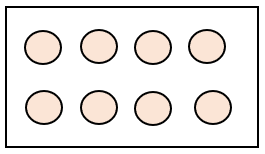Question 2.
For numbers 2a–2d, select True or False for each multiplication sentence.
a. 3 × 8 = 24
i. True
ii. False

Explanation: multiplication of 3 × 8 is 24.

Question 2.
b. 5 × 8 = 48
i. True
ii. False

Explanation: Multiplication of 5 × 8 is 40 not 48.

Question 2.
c. 7 × 8 = 56
i. True
ii. False

Explanation: Multiplication of 7 × 8 = 56.

Question 2.
d. 9 × 8 = 81
i. True
ii. False

Explanation: Multiplication of 9 × 8 is 72 not 81.

Question 3.
Peggy is putting flowers in vases. She puts either 2 or 3 flowers in each vase. If Peggy has a total of 12 flowers, how many different ways can she place them all in the vases?
Write multiplication sentences to show your work.
______ different ways

Explanation: 2 × 6 = 12; She can 2 flowers in 6 vases each or 4 × 3 = 12; she can put 3 flowers in 4 vases each or (2 × 3) + (3 × 2) = 12, she can put 2 flowers in 3 vases each and 3 flowers in 2 vases.

Question 4.
Dean plants 7 corn plants in each of 5 rows. How many corn plants does Dean plant?
______ corn plants

Answer: 7 × 5 = 35 plants

Explanation:
Given that Dean has planted 7 corn plants in each row.
The total no of rows = 5, therefore total no of plants was planted = 7 × 5 = 35.

### Test – Page 2 – Page No. 42

Question 5.
Circle groups to show 4 × (2 × 2).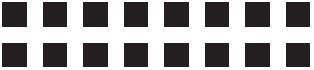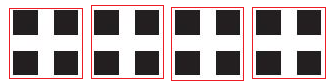Question 6.
Rebecca keeps all of her pairs of gloves in a drawer. Select the number of gloves that Rebecca could have in the drawer. Mark all that apply.
Options:
a. 5
b. 4
c. 6
d. 11
e. 12

Answer: Options b, c, and e

Explanation: Given that Rebecca kept all of her pairs of gloves in a drawer. Since it was mentioned pairs and pairs can be only in even number. Possible answers could be 4, 6, and 12.

Question 7.
Hal completed the table to describe the product of a mystery one-digit factor and each number.Part A
Give all of the possible numbers that could be Hal’s mystery one-digit factor.

Answer: 1, 3, 5, 7, 9.

Question 7.
Part B
Explain how you know that you have selected all of the correct possibilities.

Answer: The products alternate between even and odd, the mystery factor must be an odd number. We have selected all of the odd one-digit numbers.

### Test – Page 3 – Page No. 43

Question 8.
Yuri used toothpicks to make 6 separate octagons. An octagon has 8 sides. How many toothpicks did Yuri use?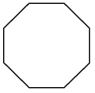______ toothpicks

Answer: 6 * 8 = 48 toothpicks.

Explanation: One octagon has 8 sides, to make one octagon Yuri need 8 toothpicks
Therefore, to make 6 octagons she needs 6*8 = 48 toothpicks.

Question 9.
Maria practiced soccer 5 days last week. She practiced 2 hours each day. How many hours did Maria practice soccer last week?
______ hours

Answer: 5 × 2 = 10 hrs

Explanation:
Given that Maria practiced for 5 days, each day she practiced for 2 hrs.
Total no of hrs she practiced in 5 days = 5 × 2 = 10 hrs.

Question 10.
Break apart the array to show 5 × 7 = (5 × 2) + (5 × 5).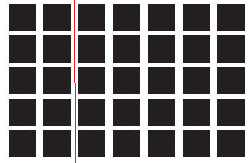Question 11.
Circle the symbol that makes the multiplication sentence true.
9 × 5(9 × 4) × 1

Answer: 9 × 5 > (9 × 4) × 1

Explanation: 9 × 5 > (9 × 4) × 1, Since 45 > (36) +1 => 45 > 37.

9 × 5(9 × 4) × 1

### Test – Page 4 – Page No. 44

Question 12.
Lori has 18 new stamps to add to her collection. She displays the stamps on pages of an album in groups of either 3, 6, or 9 stamps. How many different ways can she display the 18 new stamps?
______ different ways

Explanation:
Set 1: 3 of 6, placing 3 stamps on 6 pages each
Set 2: 6 of 3, placing 6 stamps on 3 pages each
Set 3: 2 of 9, placing 2 stamps in 9 pages each
Set 4: 1 of 3, 1 of 6, and 1 of 9, placing 1 stamp in 3 pages and 1 in 6 pages, and 1 in 9 pages each. Which in total 18 stamps
Set 5: 2 of 6 and 2 of 3, placing 2 stamps in 6 pages and 2 stamps in 3 pages each, in total 18 stamps
Set 6: 1 of 9 and 3 of 3, placing 1 stamp in 9 pages and 3 stamps in 3 pages each in total 18 stamps.
Set 7: 1 of 6 and 4 of 3, placing 1 stamp in 6 and 4 stamps in 3 pages each, in total 18 stamps.

Question 13.
A shop owner sells 3-wheel baby strollers. She checks the air in the tires on 4 different strollers. How many tires does she check in all?
Use the array to explain how you know your answer is correct.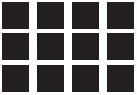Answer: 4 * 3 = 12 tires

Explanation:
Here in the array, we can group 3 rows of 4,
3 × 4 = 12. Then count by threes to check.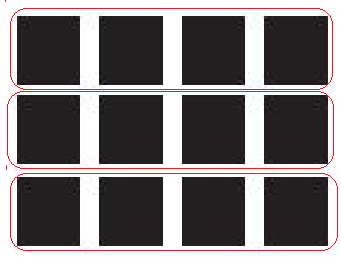Question 14.
Max arranges all of his toy cars in 9 equal rows, with 9 cars in each row. How many toy cars does Max have?
______ cars

Answer: 9 * 9 = 81 cars

Explanation:
Given that Max arranged all of his toy cars in 9 equal rows
Each row has 9 cars, therefore total no of cars = 9 * 9 = 81.

Question 15.
Deanna, Amy, and Pam pick the same number of peaches at an orchard. They each set their peaches in 4 equal piles with 6 peaches in each pile.
Write a multiplication sentence that shows how many peaches they picked.

Answer: 3 × (4 × 6) = 72

Explanation: Given that 3 persons (Deanna, Amy, and Pam) pick the same number of peaches at an orchard
Each set 4 equal piles with 6 peaches in each pile, total no of peaches per person = 4 × 6
No of peaches from all three = 3 × ( 4 × 6 ) = 72.

### Test – Page 5 – Page No. 45

Question 16.
Kate is baking 5 apple pies for the bake sale. She uses 3 red apples and 2 green apples in each pie. How many apples does Kate use? Show your work.

Explanation:
No of apple pies baked by kate = 5
In each pie she used 3 red apples and 2 green apples
So total no of apples used in a pie = 3+2 = 5
Therefore total no of apples used to make 5 apple pies = 5 ×(3+2) = 25.

Question 17.
For numbers 17a–17d, select True or False for each equation.
a. 2 × 7 = 16
i. True
ii. False

Explanation: Multiplication of 2 × 7 = 14 not 16.

Question 17.
b. 4 × 7 = 21
i. True
ii. False

Explanation: Multiplication of 4 × 7 = 28 not 21.

Question 17.
c. 6 × 7 = 42
i. True
ii. False

Explanation: Multiplication of 6 × 7 is 42.

Question 17.
d. 7 × 7 = 49
i. True
ii. False

Explanation: Multiplication of 7 × 7 is 49.

Question 18.
Circle the number that makes the multiplication sentence true.
10 ×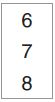= 70
______

Explanation:

10 ×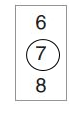= 70.

Question 19.
For numbers 19a–19d, select Yes or No to indicate whether the sum or product is equal to 9 × 4.
a. (5 × 4) + (4 × 4)
i. yes
ii. no

Explanation: 9 × 4 is 36 and (5 × 4) + (4 × 4) = (20) + (16) = 36.

Question 19.
b. 5 + (4 × 5)
i. yes
ii. no

Explanation: 9 × 4 is 36 and 5 + (4 × 5) = 25.

Question 19.
c. (3 × 3) + (2 × 2)
i. yes
ii. no

Explanation: 9 × 4 is 36 and (3 × 3) + (2 × 2) = 9 + 4 = 13.

Question 19.
d. 4 × (5 + 4)
i. yes
ii. no

Explanation: 9 × 4 is 36 and 4 × (5 + 4) = 4 × 9 = 36.

### Test – Page 6 – Page No. 46

Question 20.
A rollercoaster car can fit 6 people. How many people can fit in a rollercoaster that is 9 cars long?
______ people

Answer: 9 × 6 = 54 people.

Explanation:
Give that a rollercoaster car can fit 6 people
Number of people can fit if it having 9 cars = 9 × 6 = 54.

Question 21.
Write a multiplication sentence using the following numbers and symbols.Answer: 2 × (4 × 7) = 56.

Question 22.
Debbie started a table showing a multiplication pattern.
Part A
Complete the table. Describe a pattern you see in the products.Answer: In the given pattern it is adding 6 to the value in the next cell.

Explanation: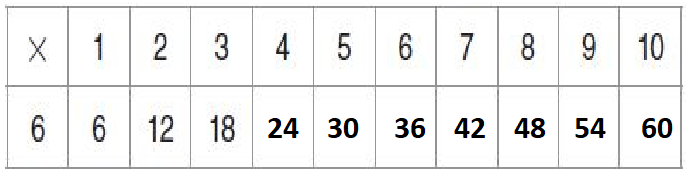Question 22.
Part B
If you multiplied 6 × 73, would the product be an even number or an odd number? Use the table to explain your reasoning.

Answer: 6 × 73 = 438.

Explanation: When any number is multiplied by 6 which is an even number the product is even, so the product of 6 × 73 = 438 is even. For example, if we take the table started by Debbie, we can see that both even and odd numbers are multiplied by 6 which even and the final result of all the products are even.Question 23.
Use the number line to show the product of 3 × 8.3 × 8 = ______

Answer: 3 × 8 = 24.

Explanation:From the number line 3 × 8 which is 8 + 8 + 8 = 24.

Conclusion:

This assessment test helps students to check their math skills. Go Math Grade 3 Chapter 10 questions are explained in detail that students can understand easily.

Scroll to Top